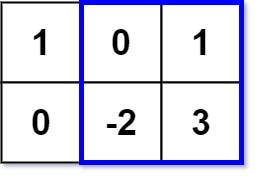363. Max Sum of Rectangle No Larger Than K
Hard
3.2K
164

Given an `m x n` matrix `matrix` and an integer `k`, return the max sum of a rectangle in the matrix such that its sum is no larger than `k`.

It is guaranteed that there will be a rectangle with a sum no larger than `k`.

Example 1:```Input: matrix = [[1,0,1],[0,-2,3]], k = 2
Output: 2
Explanation: Because the sum of the blue rectangle [[0, 1], [-2, 3]] is 2, and 2 is the max number no larger than k (k = 2).
```

Example 2:

```Input: matrix = [[2,2,-1]], k = 3
Output: 3
```

Constraints:

• `m == matrix.length`
• `n == matrix[i].length`
• `1 <= m, n <= 100`
• `-100 <= matrix[i][j] <= 100`
• `-105 <= k <= 105`

Follow up: What if the number of rows is much larger than the number of columns?

Accepted
118.3K
Submissions
268.7K
Acceptance Rate
44.0%

Seen this question in a real interview before?
1/4
Yes
No

Discussion (0)

Related Topics